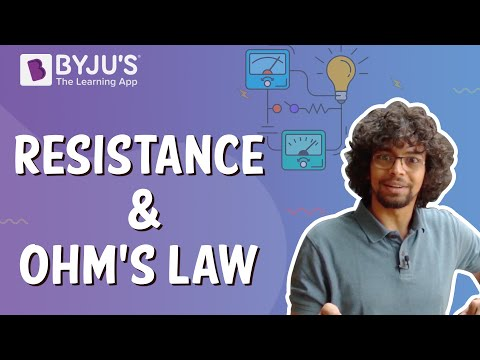# Relation Between Power And Resistance

Power in electronics is the rate of doing work. Resistance is the opposition offered against the flow of electrons. The relation between power and resistance is proportional. In physics, power and resistance can be related using two formulas. In this article, we will study both the relations between power and resistance.

## Power and Resistance

The relation between Power and Resistance can be mathematically expressed in two ways, as follows-

 P = I2R

Where,

• P is the Power measured in watt
• R is the resistance measured in ohms
• I is the electric current measured in coulombs
 $$\begin{array}{l}P=\frac{V^{2}}{R}\end{array}$$

Where,

• P is the Power measured in watt
• R is the resistance measured in ohms
• V is the electric potential measured in volts

## Power and Resistance Relation

Both the formula mentioned above gives the relation between power and resistance. Consider P = I2R the electric power is directly proportional to resistance keeping I constant. Which implies-

• When power increases, the resistance will also increase keeping current I constant.
• When Resistance decreases, Power also reduces keeping current I constant.

Consider

$$\begin{array}{l}P=\frac{V^{2}}{R}\end{array}$$
here, Power P is inversely proportional to Resistance R. which implies that-

For any constant Potential difference

• When power is high, resistance will be low.
• When power is low, the resistance will be high.

### Power and Resistance Formula

Deriving Power and Resistance formula will concrete the understanding of the concept. Electric power(P) is the measure of the electric current I with Q coulombs of charge passing through a potential difference of V volts in time t seconds. Mathematically given by-

$$\begin{array}{l}P=\frac{VQ}{t}=IV\end{array}$$
——-(1)

From Ohm’s law, we know that V=IR

Rearranging it for I we get,

$$\begin{array}{l}I=\frac{V}{R}\end{array}$$

Thus, substituting it in equation (1) we get-

$$\begin{array}{l}P=\frac{V^{2}}{R}\end{array}$$

Hope you have understood the relation between Power and Resistance, formulas along with the units of electricity and derivation. Also, power and resistance conversion in physics and electronics.

Physics Related Topics:

Stay tuned with BYJU’S for more such interesting articles. Also, register to “BYJU’S-The Learning App” for loads of interactive, engaging physics-related videos and an unlimited academic assist.

## What is Ohm’s Law?Test Your Knowledge On Relation Between Power And Resistance!